All submissions of the EM system will be redirected to Online Manuscript Submission System. Authors are requested to submit articles directly to Online Manuscript Submission System of respective journal.

# Editorial

, Volume: 6( 2)

## Accelerating Universe Explained

*Correspondence:
Bhattacharya SC , Department of Physics, A. C. College West Bengal, India, Tel: +91 9831565833; E-Mail: sunilbhattacharya@yahoo.com

Received: May 22, 2018; Accepted: May 30, 2018; Published: June 6, 2018

Citation: Bhattacharya SC. Accelerating Universe Explained. J Phys Astron. 2018; 6(2):149

### Introduction

Accelerating universe is a puzzling problem for which no explanation is available. Here I attempt to give an explanation of the phenomenon from a different angle. The explanation of course depends on some assumptions which are reasonable. The assumptions are as follows:

1) The Universe is static which may be called Einstein’s Static Universe. This assumption is made for simplicity and will be withdrawn later.

2) A photon loses energy as it passes through space. In other words photon is a decaying particle and law of decay is exponential so that,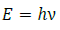, where E= energy of photon at time t which is the time of observation.

E0 = Energy of photon at time t0 which is the time of emission.

λ = decay constant,

Hence,Let Z be the Redshift then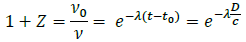D= Distance of galaxy from the observer, c= Velocity of light in vacuum, when Z is smallComparing with Hubble’s law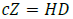where H=Hubble’s constant, we get λ=H

Thus we get-------------------------------------(A)-------------------------------------(B)

Now it may be asked how this universe will appear to an observer who believes in expanding universe but not in decay of photons. He will of course use a line element………….(1)

He will also use the relation ,where symbols have their usual meaning(2)

Comparing Equation (2) with Equation (B) it is clear that,where R is a constant

We shall call S(t) apparent expansion function

Now,……………..(C)………………(D)

Equation (C) and (D) show that this universe is expanding and accelerating.

It is very important to note that due to the decay of photons even a static universe appears expanding and accelerating.

Now a static universe is unstable. Hence we must take an expanding model with real expansion function f(t) say. Taking into account the decay of photon the apparent expansion function is,In this caseas will be shown below.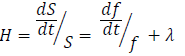Also,The first term on RHS is negative and the second and third terms are positive. If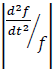is sufficiently smaller than the sum of second and third term thenwill be positive and the universe will appear accelerating though its acceleration is apparent not real.

Thus we come to the conclusion

1) Photon is a decelerating particle.

2) Acceleration of universe is apparent not real and due to the decay of photons.

3) The Universe is actually decelerating and the deceleration is small.

In fine it is interesting to notice some features of the Einstein’s static model of the Universe together with the photon decay hypothesis, which are as follows.

1) It shows that the Universe is apparently expanding.

2) It proves Hubbles law.

3) It shows Universe is accelerating apparently.

4) Acceleration is proportional to distance.

5) Constant of proportionality is H2 , where H= Hubble’s Constant

6) It gives a compact relation between redshift Z and distance

c ln(1+Z) = HD

### References

Welcome Message

###### Citations : 291

Journal of Physics & Astronomy received 291 citations as per Google Scholar report

#### Indexed In

• Open J Gate
• China National Knowledge Infrastructure (CNKI)
• Cosmos IF
• Directory of Research Journal Indexing (DRJI)
• MIAR
• Secret Search Engine Labs
• Euro Pub
• clarivate-web-of-science-logo-vector
• ICMJE

View More

Berlin, Germany

### World Congress on Quantum Physics

Berlin, Germany